The proc_freq function simulates a SAS® PROC FREQ procedure. Below is a short tutorial on the function. Like PROC FREQ, the function is both an interactive function and returns datasets.

## Create Sample Data

The first step in our tutorial is to create some sample data:

# Create sample data
text = 'x y z
6 A 60
6 A 70
2 A 100
2 B 10
3 B 67
2 C 81
3 C 63
5 C 55')

# View sample data
dat
#   x y   z
# 1 6 A  60
# 2 6 A  70
# 3 2 A 100
# 4 2 B  10
# 5 3 B  67
# 6 2 C  81
# 7 3 C  63
# 8 5 C  55

## Get Frequencies

Now that we have some data, let’s send that data to the proc_freq function to see the frequency distribution.

The options() statement below turns off printing of all procs functions. This statement is necessary so that the sample code below can pass CRAN checks. When running sample code yourself, the options statement can be omitted.

# Turn off printing for CRAN
options("procs.print" = FALSE)

# Get frequencies
proc_freq(dat, tables = y)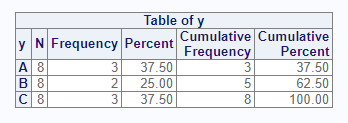The above code illustrates a one-way frequency on the “y” variable. The result shows that the “A” and “C” categories appears three times, and the “B” category appears twice. The “N” column shows that there are eight items in the population. This population is used to get the percent shown for each frequency count.

## Control Columns

The options parameter can control many aspects of the proc_freq function. For example, if you did not want the cumulative frequency and percent, you could turn off these columns with the option “nocum”.

# Turn off cumulative columns
proc_freq(dat, tables = y, options = nocum)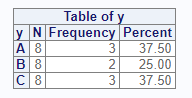Let’s say you wanted only the frequency counts, and not the other columns. This result can be achieved with the following options. Use the v() function when you are passing multiple options:

proc_freq(dat, tables = y, options = v(nocum, nonobs, nopercent))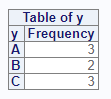## Cross Tabulation

For two-way frequencies, you can cross two variables on the tables parameter. This syntax produces a cross-tabulation table by default:

# Create crosstab
proc_freq(dat, tables = y * x)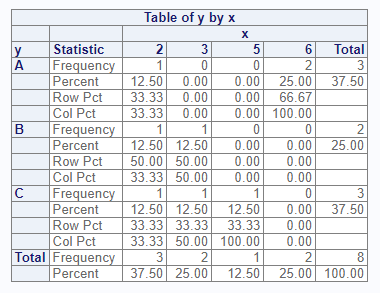## Cross Tabulation Options

If you want the data displayed in a list instead of a cross-tabulation table, you can do that with the “list” option. The “nosparse” option will turn off zero-count categories, which are included by default:

# Two-way frequency in list form
proc_freq(dat, tables = y * x, options = v(list, nosparse))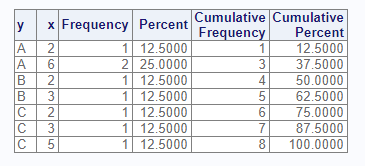The following options turn off various features of the cross-tabulation table:

# View frequencies only
proc_freq(dat, tables = y * x,
options = v(norow, nocol, nopercent))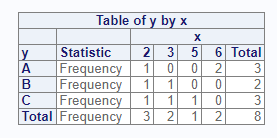## Multiple Tables

The tables parameter accepts more than one table request. To request multiple tables, pass a quoted or unquoted vector. Note that proc_freq does not accept grouping syntax, such as that allowed by SAS®. You must specify each cross-tab individually:

# Request two crosstabs
proc_freq(dat, tables = v(y * x, y * z),
options = v(norow, nocol, nopercent))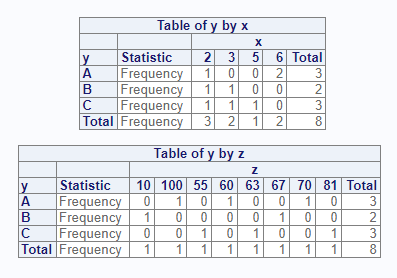## Distinct Values

The “nlevels” option can be used to count the number of distinct values in a categorical variable:

# Turn on nlevels option
proc_freq(dat, tables = y, options = nlevels)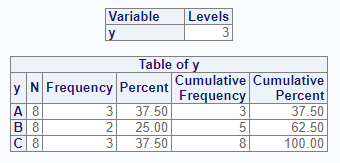## Weighted Frequencies

The weight parameter is used to achieve weighted frequencies. When a weight is specified, proc_freq will use the counts in the indicated variable for all frequency calculations.

# Add weight variable
proc_freq(dat, tables = y, weight = z)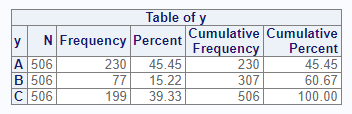## Statistics Options

The options parameter also accepts statistics options. For two-way tables, you may request either Chi-Square or Fisher’s tests of association. Here is an example of the Chi-Square test:

# Request Chi-Square and Output datasets
res <- proc_freq(dat, tables = y * x, options = chisq)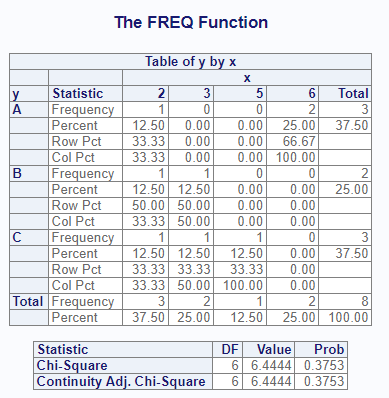# View results
res
# $y * x # VAR1 VAR2 CAT1 CAT2 N CNT PCT # 1 y x A 2 8 1 12.5 # 2 y x A 3 8 0 0.0 # 3 y x A 5 8 0 0.0 # 4 y x A 6 8 2 25.0 # 5 y x B 2 8 1 12.5 # 6 y x B 3 8 1 12.5 # 7 y x B 5 8 0 0.0 # 8 y x B 6 8 0 0.0 # 9 y x C 2 8 1 12.5 # 10 y x C 3 8 1 12.5 # 11 y x C 5 8 1 12.5 # 12 y x C 6 8 0 0.0 # #$chisq:y * x
#      CHISQ CHISQ.DF   CHISQ.P
# 1 6.444444        6 0.3752853

## Output Datasets

You may control datasets returned from the proc_freq function using the output parameter. This parameter takes three basic values: “out”, “report”, and “none”. The “out” keyword requests datasets meant for output, and is the default. These datasets have standardized column names, and sometimes have additional columns to help with data manipulation. The “report” keyword requests the exact datasets used to create the interactive report. For both keywords, if there is more than one dataset, they will be returned as a list of datasets. The name of the list item will identify the dataset. You may specify the names of the output tables in the list by using a named table request.

Here is an example of the “out” option:

# Request output data
res <- proc_freq(dat, tables = v(x, y, MyCross = y * x),
output = out)

# View results
res
$x VAR CAT N CNT PCT 1 x 2 8 3 37.5 2 x 3 8 2 25.0 3 x 5 8 1 12.5 4 x 6 8 2 25.0$y
VAR CAT N CNT  PCT
1   y   A 8   3 37.5
2   y   B 8   2 25.0
3   y   C 8   3 37.5

\$MyCross
VAR1 VAR2 CAT1 CAT2 N CNT  PCT
1     y    x    A    2 8   1 12.5
2     y    x    A    3 8   0  0.0
3     y    x    A    5 8   0  0.0
4     y    x    A    6 8   2 25.0
5     y    x    B    2 8   1 12.5
6     y    x    B    3 8   1 12.5
7     y    x    B    5 8   0  0.0
8     y    x    B    6 8   0  0.0
9     y    x    C    2 8   1 12.5
10    y    x    C    3 8   1 12.5
11    y    x    C    5 8   1 12.5
12    y    x    C    6 8   0  0.0

Notice that the way output datasets are requested from the proc_freq function is much simpler than the corresponding mechanism in SAS®. With proc_freq, by default, all requested tables and statistics will be returned in a list. No other output parameters are needed.

## Data Shaping

The proc_freq function provides three options for shaping data: “wide”, “long”, and “stacked”. These options control how the output data is organized. The options are also passed on the output parameter. The shaping options are best illustrated by an example:

# Shape wide
res1 <- proc_freq(dat, tables = y,
output = wide)

# Wide results
res1
#   VAR CAT N CNT  PCT
# 1   y   A 8   3 37.5
# 2   y   B 8   2 25.0
# 3   y   C 8   3 37.5

# Shape long
res2 <- proc_freq(dat, tables = y,
output = long)

# Long results
res2
#   VAR STAT    A  B    C
# 1   y    N  8.0  8  8.0
# 2   y  CNT  3.0  2  3.0
# 3   y  PCT 37.5 25 37.5

# Shape stacked
res3 <- proc_freq(dat, tables = y,
output = stacked)

# Stacked results
res3
#   VAR CAT STAT VALUES
# 1   y   A    N    8.0
# 2   y   A  CNT    3.0
# 3   y   A  PCT   37.5
# 4   y   B    N    8.0
# 5   y   B  CNT    2.0
# 6   y   B  PCT   25.0
# 7   y   C    N    8.0
# 8   y   C  CNT    3.0
# 9   y   C  PCT   37.5

As seen above, the “wide” option places the statistics in columns across the top of the dataset and the categories in rows. This shaping option is the default. The “long” option places the statistics in rows, with each category in columns. The “stacked” option places both the statistics and the categories in rows.

These shaping options reduce some of the manipulation needed to get your data in the desired form. These options were added for convenience during the development of the procs package, and have no equivalent in SAS®.

Next: The Means Function